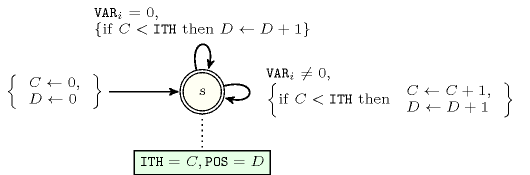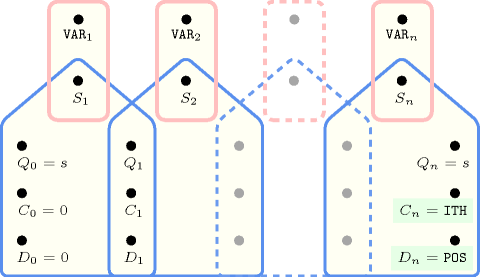## 5.203. ith_pos_different_from_0

Origin

N. Beldiceanu

Constraint

$\mathrm{𝚒𝚝𝚑}_\mathrm{𝚙𝚘𝚜}_\mathrm{𝚍𝚒𝚏𝚏𝚎𝚛𝚎𝚗𝚝}_\mathrm{𝚏𝚛𝚘𝚖}_\mathtt{0}\left(\mathrm{𝙸𝚃𝙷},\mathrm{𝙿𝙾𝚂},\mathrm{𝚅𝙰𝚁𝙸𝙰𝙱𝙻𝙴𝚂}\right)$

Arguments
 $\mathrm{𝙸𝚃𝙷}$ $\mathrm{𝚒𝚗𝚝}$ $\mathrm{𝙿𝙾𝚂}$ $\mathrm{𝚍𝚟𝚊𝚛}$ $\mathrm{𝚅𝙰𝚁𝙸𝙰𝙱𝙻𝙴𝚂}$ $\mathrm{𝚌𝚘𝚕𝚕𝚎𝚌𝚝𝚒𝚘𝚗}\left(\mathrm{𝚟𝚊𝚛}-\mathrm{𝚍𝚟𝚊𝚛}\right)$
Restrictions
 $\mathrm{𝙸𝚃𝙷}\ge 1$ $\mathrm{𝙸𝚃𝙷}\le |\mathrm{𝚅𝙰𝚁𝙸𝙰𝙱𝙻𝙴𝚂}|$ $\mathrm{𝙿𝙾𝚂}\ge \mathrm{𝙸𝚃𝙷}$ $\mathrm{𝙿𝙾𝚂}\le |\mathrm{𝚅𝙰𝚁𝙸𝙰𝙱𝙻𝙴𝚂}|$ $\mathrm{𝚛𝚎𝚚𝚞𝚒𝚛𝚎𝚍}$$\left(\mathrm{𝚅𝙰𝚁𝙸𝙰𝙱𝙻𝙴𝚂},\mathrm{𝚟𝚊𝚛}\right)$
Purpose

$\mathrm{𝙿𝙾𝚂}$ is the position of the ${\mathrm{𝙸𝚃𝙷}}^{th}$ non-zero item of the sequence of variables $\mathrm{𝚅𝙰𝚁𝙸𝙰𝙱𝙻𝙴𝚂}$.

Example
$\left(2,4,〈3,0,0,8,6〉\right)$

The $\mathrm{𝚒𝚝𝚑}_\mathrm{𝚙𝚘𝚜}_\mathrm{𝚍𝚒𝚏𝚏𝚎𝚛𝚎𝚗𝚝}_\mathrm{𝚏𝚛𝚘𝚖}_\mathtt{0}$ constraint holds since 4 corresponds to the position of the 2th non-zero item of the sequence $30086$.

Typical
 $|\mathrm{𝚅𝙰𝚁𝙸𝙰𝙱𝙻𝙴𝚂}|>1$ $\mathrm{𝚛𝚊𝚗𝚐𝚎}$$\left(\mathrm{𝚅𝙰𝚁𝙸𝙰𝙱𝙻𝙴𝚂}.\mathrm{𝚟𝚊𝚛}\right)>1$ $\mathrm{𝚊𝚝𝚕𝚎𝚊𝚜𝚝}$$\left(1,\mathrm{𝚅𝙰𝚁𝙸𝙰𝙱𝙻𝙴𝚂},0\right)$
Symmetry

An occurrence of a value of $\mathrm{𝚅𝙰𝚁𝙸𝙰𝙱𝙻𝙴𝚂}.\mathrm{𝚟𝚊𝚛}$ that is different from 0 can be replaced by any other value that is also different from 0.

Arg. properties

Suffix-extensible wrt. $\mathrm{𝚅𝙰𝚁𝙸𝙰𝙱𝙻𝙴𝚂}$.

Keywords
Automaton

Figure 5.203.1 depicts the automaton associated with the $\mathrm{𝚒𝚝𝚑}_\mathrm{𝚙𝚘𝚜}_\mathrm{𝚍𝚒𝚏𝚏𝚎𝚛𝚎𝚗𝚝}_\mathrm{𝚏𝚛𝚘𝚖}_\mathtt{0}$ constraint. To each variable ${\mathrm{𝚅𝙰𝚁}}_{i}$ of the collection $\mathrm{𝚅𝙰𝚁𝙸𝙰𝙱𝙻𝙴𝚂}$ corresponds a 0-1 signature variable ${S}_{i}$. The following signature constraint links ${\mathrm{𝚅𝙰𝚁}}_{i}$ and ${S}_{i}$: ${\mathrm{𝚅𝙰𝚁}}_{i}=0⇔{S}_{i}$.

##### Figure 5.203.1. Automaton of the $\mathrm{𝚒𝚝𝚑}_\mathrm{𝚙𝚘𝚜}_\mathrm{𝚍𝚒𝚏𝚏𝚎𝚛𝚎𝚗𝚝}_\mathrm{𝚏𝚛𝚘𝚖}_\mathtt{0}$ constraint##### Figure 5.203.2. Hypergraph of the reformulation corresponding to the automaton of the $\mathrm{𝚒𝚝𝚑}_\mathrm{𝚙𝚘𝚜}_\mathrm{𝚍𝚒𝚏𝚏𝚎𝚛𝚎𝚗𝚝}_\mathrm{𝚏𝚛𝚘𝚖}_\mathtt{0}$ constraint